Achieve Innovative Process Improvement＋Standardization by IT System with MS 365.# What is Standard Deviation? There are too many Excel functions for Standard Deviation!\ この記事を共有 /
What is Standard Deviation? There a...

This article explains ‘What Standard Deviation is’, ‘Why it’s needed’, and differences between ‘Population’ and ‘Sample’. Also, there are several Standard Deviation Excel functions. You can learn which one you should use.

(Duration: 8:52)

＜＜ Related Posts ＞＞

## What is Deviation (Average – Measured Data)?

Hi, this is Mike Negami, Lean Sigma Black Belt.

One of my viewers sent me an email asking:“Can you make videos regarding Sigma Value and examples of its calculations?”

Thank you, Reham for the request. We often talk about “Six Sigma”, but do we really understand Sigma? Sigma means Standard Deviation in statistics. So, does “Six Sigma” mean 6 times the Standard Deviation? Today, we’ll focus on studying Sigma.

Let’s say that we measured 5 parts’ length as samples from your production line yesterday and today. What can we do to compare yesterday’s and today’s results? You would calculate and compare their averages first. Add all the data values and divide by the number of data. One little issue happened here. Even though the measured data are totally different, the averages of yesterday and today were exactly the same. Here is that situation on a chart. Today’s data seems to have more variation. (See the chart below.)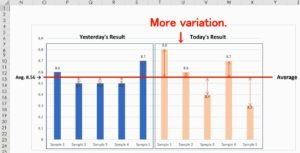The numerical value of this variation is Standard Deviation. In Lean Sigma, it’s fundamental to minimize variations and keep the quality consistent, therefore this Standard Deviation is very important.  Well, how can we calculate this variation? The first thing is to add all the subtractions from each number to the average. But since those subtractions are plus and minus, their total will always be zero. Unfortunately, this is not useful.

The subtraction from the average is called deviation [=Average – Measured Data], and there are two ways to make the deviation always positive.  One is to use the absolute values of those deviations, which are each number’s distance from their average, add them all and divide it by the number of the data. The other way is to square the deviation.

## Standard Deviation = Square Root of the Variance.  Why using the Square Root?

To square the deviation [=Deviation^2] will always be positive.  The value after adding them all and dividing the total by the number of the data is called variance [=SUM(Deviations)/the # of Data], which is often used in statistics. I will not go into detail at this time, but since using variance made more sense, that has been adopted in statistics. Yesterday’s variance was 0.006 and today was 0.034.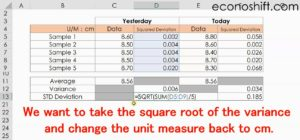But since variance was squared, its unit measure has changed. This time it has changed from cm to square cm.   Therefore, we want to take the square root of the variance [=SQRT(Variance)] and change the unit measure back to cm, and this is Standard Deviation.  Yesterday’s Standard Deviation was 0.08 cm and today’s was 0.185 cm, so it was numerically clarified that we had a bigger variation today.

## There are several Standard Deviation functions.  Which one should I use?

Do you understand the concept of Standard Deviation well? If so, let ‘s have Excel do the rest of the calculations.

In Excel, type , which stands for Standard Deviation, then you’ll see there are several Standard Deviation functions. If you are using Excel 2010 or newer, use the STDEV.P function or the STDEV.S function.  With an earlier version, use the STDEVP or STDEV.  The last digit, P, of the STDEV.P function stands for Population, and here it also says “based on the entire population”.The other one, the STDEV.S Function’s S stands for Sample and here it says, “based on a sample”.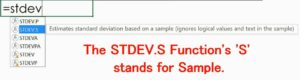## What’s difference between ‘Population’ and ‘Sample’?

These two differences also make Standard Deviation difficult. This time, we have 5 data each day, but in practice we would make lots of parts. For example, let’s say you make 100 parts every day, if you measure all parts’ lengths accurately and calculate the Standard Deviation, it’s really accurate. We call that 100% inspection, but it takes time and cost.

Therefore, we do sampling inspection in which we randomly take samples and consider them to reflect their population. STDEV.S will be used in this case and STDEV.P will be used with 100% inspection.  By the way, using the STDEV.P function got the same result as the first calculation. With the STDEV.S Function, yesterday’s result was 0.089 and today’s was 0.207, which means both the variations show a slight increase.

With this function, when calculating variance, the total of squared deviations is divided by the number of data minus 1 instead of by just the number of data.Since it divides by a smaller number, the result will be a little bigger. Meanwhile, it’s easy to imagine that since the population is much bigger than its samples, the population’s variation is larger than the sample one.  Although there are a few statistical reasons, basically past wise people decided that when calculating Standard Deviation from samples, we should use the number of data minus 1.  In summary, remember to use STDEV.S with samples, unless it’s for 100% inspections.

## What are ‘Sigma’, ‘Sigma Value in a Process’ and ‘the Origin of Six Sigma’?

At last we can talk about the initial question regarding Sigma Value.  For example, today’s data’s 3 Sigma is 3 x Standard Deviation, which is 0.621 cm.  But when asked what the 3 Sigma Value of this process is, it’s the average ±3 Sigma = 8.56 ± 0.621, which is between 7.939 and 9.181.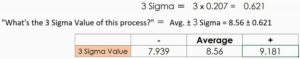This is generally used as the upper and lower control limits when writing a control chart.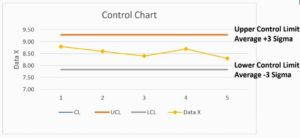By the way, the name “Six Sigma” that Motorola developed comes from the aim of 6 Sigma level quality. However, its concept is quite different from the above-mentioned Sigma Value.  The 6 Sigma value is the average ± 6 sigma, so it’s easier to aim between 7.318 to 9.802 cm rather than aiming at 3 Sigma Value. Some people may get confused by that.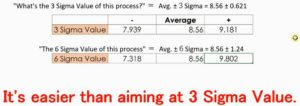The original meaning of “Six Sigma” is to improve your process with enough Sigma, which is Standard Deviation, to reach 6 for the Z score, which is another measure in statistics.

The Z Score = the smaller one of (Upper Control Limit – Average) / Std Deviation or (Average – Lower Control Limit) / Std Deviation

The level in which it’s achieved is “only 3.4 defects per 1 million pieces” which is well-known. Therefore, it’s nothing to do with 6 times Sigma. Does this make sense?

Today we discussed various things about Sigma and Standard Deviation which are very important in Quality Control.

＜＜ Related Posts ＞＞

### 最新記事